# Investigation of Effective Parameters on the Performance of the Helium Liquefaction Cycle

Investigation of Effective Parameters on the Performance of the Helium Liquefaction Cycle
Center of Excellence in Energy Conversion (CEEC), Department of Mechanical Engineering, Sharif University of Technology, Tehran 11155-1639, Iran

Corresponding Author Email:
afshin@sharif.edu
Page:
1009-1018
|
DOI:
https://doi.org/10.18280/ijht.370410
10 November 2018
|
Accepted:
19 December 2019
|
Published:
26 December 2019
| Citation

OPEN ACCESS

Abstract:

Due to its unique properties, helium has wide application in different industries and scientific fields, which has turned it into a strategic material. Helium liquefaction plants include wide temperature range from 300 k to 4.2 k, so these plants have high energy consumption. A lot of studies have done to optimize the operation of these cycles. In this research, an exergy analysis is performed for a liquid helium production plant. The optimal performance of 3 and 4 stage cycles is extracted using parametric study and the results are compared with those of Collins dual-expander cycle. The results show that by increasing the number of cooling stages, not only the compressor optimum discharge pressure is reduced, but also the cycle efficiency dramatically increases and the power consumption of the cycle decreases. Further, a sensitivity analysis of the exergy efficiency of the 2, 3 and 4 expander cycles is compared to the heat exchangers effectiveness, expander’s efficiency and the input flow rate to the expanders.

Keywords:

cold box, cryogenic process, cycle efficiency, exergy analysis, helium liquefaction, parametric study

1. Introduction

Due to its unique and various properties, e.g. low liquid-state viscosity, high conductivity coefficient, non-reactivity and being liquid at about 4.2 K, helium has wide application in different industries such as medicine, surgery, imaging, transportation and electronic equipment. Currently, natural gas is the only economic source of liquid helium production. In the last century, for the extraction and liquefaction of helium, different cycles have been designed and built based on regional conditions. The purpose of these researches is to enhance liquefaction and reliability, and reduce energy consumption. The helium liquefaction unit includes various equipment, e.g. compressors, heat exchangers and expanders.

Collins was the first researchers who designed an economical helium liquefier [1, 2]. In this cycle a J-T valve and two expanders were used to liquefy helium. The analysis of Collins cycle as a basis cycle for today's liquefaction cycles can be a great help for the analysis of more complex cycles. Liquefaction cycles can be analyzed by two methods: The first one is to use the first law of thermodynamics for cycle optimization. This law is exclusively useful for the problems, in which energy conservation is examined [3-5]. The second method is to utilize exergy analysis, which is based on the second law of thermodynamics. Using this method provides adequate information on the quality of flow at any point in the cycle and so it’s more suitable for analyzing this type of problems. In fact, expressing the flow properties as exergy, integrates the expression of flow properties at different point in the cycle, thereby simplifying their comparison.

Considerable efforts have been made to study the effect of different thermodynamic parameters of liquefaction cycles, e.g. compressors discharge pressure, number of compression stages and intermediate pressures, total input flow rates to expanders and flow distribution between them.

In the study based on thermodynamic analysis (first law), Attery investigated the effect of distributing Collin cycle expander flow rate on cycle efficiency and showed that the energy required by this cycle for optimal performance depended on this parameter . Using exergy analysis, Treep discussed the exergy losses in equipment of liquefier cycle , which formed the basis of liquefier optimization because it determined where the input energy and exergy of the cycle was destroyed. According to this study, approximately half of the exergy entering the cycle lost at the compression stage.

Following their previous research, Thomas et al. first determined the portion of exergy losses in each equipment for Collins dual-expander cycle and then investigate the effects of various performance parameters on cycle exergy efficiency . They studied the effects of various parameters including compressor discharge pressure, expanders total input flow rate and flow distribution between them, expanders efficiency, effectiveness of heat exchangers, number of cooling stages used in the cycle and type of the cold end on the level of liquefaction and cycle efficiency . By varying different parameters of the cycle such as expander efficiency, they attempted to show the trend of changes in cycle efficiency and the exergy losses of different equipment.

According to their studies on Collins dual-expander cycle, cycle efficiency is maximum at the pressure of 22 bar. By studying the effect of input flow rate to the expanders, they suggested that efficiency was maximum when assigning 80% of the compressors output flow rate equally to the expanders at any pressure. Moreover, as expected, cycle efficiency was maximized by assigning 80% flow rate equally to the expanders at the pressure of 22 bar .

In another study, Thomas et al. investigated the effect of increasing the number of cooling stages and putting intermediate pressure in structure of the cycle . Accordingly, increasing the number of cooling stages decreased exergy loss in the heat exchangers by reducing their performance temperature range and, thereby, decreasing the input temperature to the J-T valve which, in turn, enhanced cycle efficiency. Also, by making more consistency between the heat capacities of the heat exchangers flow and reducing the expanders losses, intermediate pressure enhanced the cycle efficiency .

Further studies have been performed on the cycles with more expanders and cooling stages [11-14].

In addition to the research on the helium liquefaction cycle, further studies have been conducted on the analysis and optimization of thermodynamic parameters in liquefaction cycles for other gases [15-17]. Thomas et al. examined the effects of different parameters on cycle efficiency and attempted to identify the important parameters in designing an optimal cycle and propose a general trend for analyzing and optimizing large-scale industrial cycles . However, all their studies on different cycles were conducted at the compressor discharge pressure of 22 bar, while this pressure was optimal only for Collins dual-expander cycle. Consequently, they did not compare different cycles at their optimal pressures and, thus, their optimal performance states.

In the present research, by varying compressor discharge pressure in 3 and 4 expander cycles, first, the optimal pressure is calculated in each state. Then, through the parametric analysis of heat exchangers effectiveness, expanders efficiency, expanders total input flow rates, etc., the effects of these parameters on cycle optimal pressure are indicated and, finally, the optimal performance state of every cycle is determined.

2. Solution Method and Governing Equations

Figure 1 shows the schematic representation of a liquefaction cycle with n-cooling stages. Since the purpose of this research is to study 3 and 4 expander cycles, the value of n is equal to 3 and 4. Conventional helium liquefaction cycles include three units:

(1) Compression unit: This unit responsible for conducting a compression process near the constant temperature line, which includes several stages together with inter and after coolers;

(2) Pre-cooling unit: This unit which comprises different cooling stages (each stage includes an expander and two heat exchangers) is responsible for reducing the temperature of high-pressure helium flow below the maximum inversion temperature of helium.

(3) Liquefaction unit: in this unit, a fraction of gas flow is converted into liquid through constant-enthalpy or constant-entropy expansion. This unit is known as cold end.

In this research, the following assumptions are considered for analyzing and obtaining the equations:

(1) The system is operating in the steady state.

(2) The compressors and expanders efficiency do not change with pressure, temperature or flow rate.

(3) The heat transfer coefficient of heat exchangers does not change with pressure, temperature or flow rate.

(4) The effects of heat leakage in the heat exchangers and other irreversibilities, e.g. longitudinal heat conduction and flow maldistribution, are all considered in the effective UA of heat exchangers.

(5) The effects of heat leak into the pipelines have been neglected.

## 1.jpgFigure 1. Schematic of the n-expander liquefaction cycle

The 32-parameter modified BWR equation of state has been used for determining the thermodynamic properties of helium . Also, the exergy balance equation was utilized for obtaining the cold box exergy efficiency, cycle efficiency and liquefaction:

$\sum_{\mathrm{j}} \mathrm{Q}^._{j}\left(1-\frac{\mathrm{T}_{0}}{\mathrm{T}_{\mathrm{j}}}\right)-\dot{\mathrm{W}}+\sum_{\mathrm{in}} \mathrm{E}^. \mathrm{x}_{\mathrm{flow}}$$-\sum_{\mathrm{out}} \mathrm{{E}^.x}_{\mathrm{flow}}-\mathrm{Ex}_{\mathrm{dest}}=0 (1) The first term shows the exergy transferred by heat transfer; \dot{\mathrm{Q}}_{\mathrm{j}} is the heat transfer rate from the control volume boundary (kW); T_{j} shows the temperature of each equipment (K); and T_0 indicates the temperature of reference condition (K). The second terms show the exergy transferred as a work in control volume and is equal to the electrical and mechanical works that transfer to the system (kW). The third and fourth terms denote the input/output exergy to/from the control volume by mass flow rate (kW), is calculated by Eq. (2): \dot{\mathrm{E}} \mathrm{x}_{\mathrm{flow}}=\dot{\mathrm{m}} \times \psi (2) where, m ̇ is the mass flow rate (kg s-1) and ψ indicates the exergy of flow per unit of mass (kJ.kg-1). Eq. (3). depicts the calculation of ψ, in which h and s respectively denote the specific enthalpy and entropy of the fluid: \psi=\left[\left(\mathrm{h}-\mathrm{h}_{0}\right)-\mathrm{T}_{0}\left(\mathrm{s}-\mathrm{s}_{0}\right)\right] (3) where, h_0 and s_0 are the specific enthalpy and entropy of the fluid at the temperature and pressure of reference condition, respectively. In this study, T_{0}=300 \mathrm{K} and P_{0}=1.013 bar. The fifth term in Eq. (1). shows the thermodynamic irreversibility’s in the cycle that is calculated by Eq. (4).: \dot{\mathrm{E}} \mathrm{x}_{\mathrm{dest}}=\mathrm{T}_{0} \Delta^. \mathrm{S}_{\mathrm{g}} (4) where, \Delta^. S_{g} indicates the entropy generation rate (kW K-1) in the process. By applying the exergy balance equation to C.V. (Figure 1), the following equation is obtained: \mathrm{\dot{m}}\left(\psi_{2}-\psi_{1^{\prime}}\right)=\dot{\mathrm{m}}_{l}\left(\psi_{l}-\psi_{1^{\prime}}\right)+\sum_{\mathrm{i}=1}^{\mathrm{n}} \dot{\mathrm{m}}_{\mathrm{expn}} \Delta \psi_{\mathrm{expn}}$$+ {\dot{E}{x}_{dest-coldbox}}$                                  (5)

The mass flow rate balance equation in Figure 1 will be as follow:

$\dot{\mathrm{m}}=\dot{\mathrm{m}}_{l}+\dot{\mathrm{m}}_{l p}$                                   (6)

where, $\dot{m}_{L}$ shows the output liquid flow rate of the cycle and $\dot{\mathrm{m}}_{\mathrm{LP}}$ indicates the flow rate of helium on the low-pressure line. The following equation is obtained by Eq. (5).:

$\frac{\dot{\mathrm{m}_{l}}}{\dot{\mathrm{m}}}\left(\psi_{l}-\psi_{1^{\prime}}\right)=\left(\psi_{2}-\psi_{1^{\prime}}\right)-$$\sum_{i=1}^{n} \frac{\dot{m}_{e x p n}}{\dot{m}} \Delta \psi_{e x p n}-\frac{ { {E^.x}_ {dest-coldbox} }}{\dot{m}}=0$                     (7)

The output liquid rate can be written in the following form based on exergy equations:

$\mathrm{y}=\frac{\dot{\mathrm{m}}_{1}}{\dot{\mathrm{m}}}=\frac{\left(\psi_{2}-\psi_{1} \prime\right)}{\left(\psi_{1}-\psi_{1} \prime\right)}-\sum_{\mathrm{i}=1}^{\mathrm{n}} \frac{\dot{\mathrm{m}}_{\mathrm{expn}}}{\dot{\mathrm{m}}} \frac{\Delta \psi_{\mathrm{expn}}}{\left(\psi_{1}-\psi_{1} \prime\right)}$                       (8)

Since the expanders are rarely used in conventional small-scale cycles, Eq. (9)., and Eq. (10). demonstrate the input exergy rate to and the output exergy rate from the cold box, respectively:

Net exergy input $=\dot{\mathrm{m}}\left(\psi_{2}-\psi_{1^{\prime}}\right)$                       (9)

Net exergy Output $=\dot{\mathrm{m}}_{l}\left(\psi_{l}-\psi_{1^{\prime}}\right)$                         (10)

The cold box exergy efficiency, which indicates the ability of the cycle for liquefaction, can be defined based on Eq. (7). as:

$\eta_{\mathrm{ex}-\mathrm{coldbox}}=\frac{\dot{\mathrm{m}}_{l}\left(\psi_{l}-\psi_{1} \prime\right)}{\dot{\mathrm{m}}\left(\psi_{2}-\psi_{1} \prime\right)} \times 100 \%$                               (11)

Eq. (12). indicates the compressor exergy efficiency:

$\eta_{\mathrm{ex}-\mathrm{comp}}=\frac{\dot{\mathrm{m}}\left(\psi_{2}-\psi_{1} \prime\right)}{\dot{\mathrm{W}}_{\mathrm{comp}}} \times 100 \%$              (12)

The numerator of the above fraction is the input exergy to the cycle and its denominator is the total input electrical work to the compressors.

Furthermore, the cycle exergy efficiency, which includes the exergy efficiencies of the cold box and compressor, is calculated based on Eq. (13).:

$\eta_{\text {ex-cycle }}=\eta_{\text {ex-coldbox }} \times \eta_{\text {ex-comp }}=\frac{\dot{\mathrm{m}}_{l}\left(\psi_{l}-\psi_{1}^{\prime}\right)}{\dot{\mathrm{W}}_{\text {comp }}} \times 100 \%$          (13)

The non-dimensional heat transfer coefficient of heat exchangers is defined according to the equation below:

$(\mathrm{UA})^{*}=\frac{\mathrm{UA}}{\mathrm{\dot{m}c}_{\mathrm{p}}}$                                (14)

Here, the specific heat capacity $c_p$ is measured at temperature and pressure of compressor suction flow.

3. Results and Discussions

The home-made code is used to simulate and obtain the results. In all the simulation states, the efficiency of heat exchangers and expanders is assumed 97% and 70%, respectively and the flow distribution between the expanders is assumed equal, unless note otherwise.

3.1 Effect of cooling stages

Generally, increasing the number of cooling stages reduces the heat exchangers temperature range and also input temperature to the J-T valve, thereby enhancing cycle efficiency and liquefaction level. Assuming the high pressure of the cycle is 20 bar and low pressure of the cycle is 1.01 bar, Figure 2 indicates the effect of increasing cooling stages on the cold box exergy efficiency and input temperature to the J-T valve. The following results are achieved from Figure 2.

## 2.jpg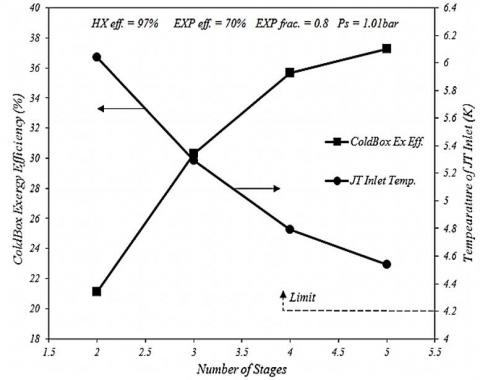Figure 2. Changes in cold box exergy efficiency based on the number of pre-cooling stages in the liquefaction cycle at the compressor discharge pressure of 20 bar

• Increasing the number of cooling stages enhance its cold box exergy efficiency from 22% (dual-expander cycle) to 36% (4 expander cycles).
• Based on Figure 2, comparing with other previous case study on parameters, increasing the number of pre-cooling stages have a greater effect on the cycle efficiency. Thomas indicated that the cold box exergy efficiency of Collins dual-expander cycle increased by 4% with increasing the efficiency of heat exchangers from 97% to 99% and cold box exergy efficiency of this cycle increased by 2% with increasing the efficiency of expanders from 70% to 75%. However, by increasing the number of cooling stages from 2 to 3, the cold box exergy efficiency increased by 8%.
• As already note, increasing the number of pre-cooling stages from 2 to 3 enhance the cold box exergy efficiency up to 8%, while increasing cooling stages from 3 to 4 expanders caused 6% improvement in the cold box exergy efficiency. By adding a further pre-cooling stage to the cycle structure, it is revealed that any further addition saturated the cycle in terms of the increase in exergy efficiency because the input temperature to J-T valve could not be less than a determined level, i.e. helium gas saturation temperature. It should be noted that exergy efficiency saturation of the helium liquefaction cycle at four pre-cooling stages is discussed for an ideal cycle because the ideal assumption’s, e.g. ignoring the pressure drop and heat leak in different cycle parts, are conducted. Since in reality, these assumptions do not exactly hold, it should be investigated when the cycle exergy efficiency reaches saturation in real conditions.

3.2 Effect of compressor discharge pressure

Figure 3, Figure 4 and Figure 5 show the effect of variation in compressor discharge pressure on the cold box exergy efficiency, cycle exergy efficiency and level of liquefaction for 2, 3 and 4 expander cycles, respectively. In liquefier cycle, the optimal operating pressure of a liquefaction cycle is an important parameter since it shows the cycle power consumption. Results of this study show:

• The chart of the cold box exergy efficiency based on discharge pressure in 3 and 4 expander cycles have optimal points like Collins dual-expander cycle. The optimal pressure of 2, 3 and 4 expander cycles are 20, 16 and 14 bar, respectively.
• As the number of pre-cooling stages increase, its optimal operating pressure is decreased. This reduction does not have linear trend and it seems converged to a constant state similar to the cold box exergy efficiency and the input temperature to the J-T valve.

## 3.jpg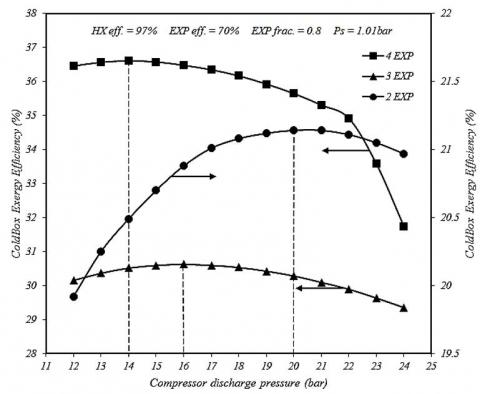Figure 3. Changes in the cold box exergy efficiency for 2, 3 and 4 pre-cooling stages in the liquefaction cycle based on the compressor discharge pressure

• The cold box exergy efficiency in the 4 expanders cycle has a sudden reduction at high pressures, which is due to the properties of helium at low temperatures and­ high pressures, because the 4 expander cycles greatly decreased the input temperature to the J-T valve at high pressure. Based on helium properties in this condition e.g., at low temperatures and high pressures, the liquefaction of helium decreased due to the throttling process.
• Increasing the stages from 2 to 3 enhance the level of liquefaction by 2%, while increasing the stages from 3 to 4 enhance the liquefaction by 1.5% at the cycle optimal point. In the 4 expanders cycle, the liquefaction level could reach 10% at the pressure of 22 bar, but it must be noted this pressure isn’t the cycle optimal pressure.

## 4.jpg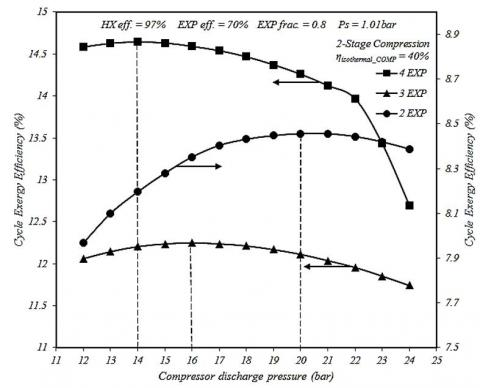Figure 4. Changes in the cycle exergy efficiency for 2, 3 and 4 pre-cooling stages in the liquefaction cycle based on the compressor discharge pressure

## 5.jpgFigure 5. Changes in the liquefaction level for 2, 3 and 4 pre-cooling stages of the liquefaction cycle based on the compressor discharge pressure

Furthermore, the exergy destruction in various equipment of 2, 3 and 4 expander liquefaction cycles are drawn based on total input exergy to the cold box in Figure 6 with the following results:

• Total exergy destruction between different equipment of the liquefaction cycle decreased by 15% from the 2 to 4 expander optimal cycles.
• The highest exergy destruction between the equipment of the liquefaction cycle occurred in the expanders. As the number of pre-cooling stages increase, exergy destruction in expanders decrease, because the average input temperature to the expanders is enhanced by increasing the number of pre-cooling stages. This result could be proven based on thermodynamic equations.
• After the expanders, the highest exergy destruction occurred in heat exchangers. As already mentioned, increasing the number of pre-cooling stages reduce the temperature ranges in the heat exchangers, thereby exergy destruction in cold box is reduced.

The ratio of exergy destruction to the input exergy of J-T valve is significant due to the small amount of J-T valve flow rate.

## 6.jpgFigure 6. Exergy destruction in different equipment of the liquefaction cycle to the total input exergy to the cold box at the cycle optimal performance

3.3 Effect of total input flow rate to the expanders

According to Thomas, the highest exergy efficiency of the cold box in Collins dual-expander cycle occurred when the expanders total input flow rates equaled 80% of the input flow rate to the cold box [4, 8]. Figure 7 and Figure 8 demonstrate the results of investigating the effect of this parameter on the efficiency of the 3 and 4 expander cycles, respectively. Based on these two figures, it is found that:

## 7.jpgFigure 7. Changes in the cold box exergy efficiency for various input flow rate to the expanders based on the compressor discharge pressure in the 3 expanders cycle

• The highest exergy efficiency of the cold box occurs in the 3 and 4 expander cycles for the expanders mass flow rate ratio of 80%. Afterwards, in 3 expander cycles, 70% flow rate ratio cause higher efficiency than 90% flow rate ratio at the cycle optimal pressure point. Nevertheless, for the 4 expanders cycle, higher efficiency is achieved at 90% flow rate ratio than 70%.
• It is clear that there is specific optimal pressure for the cycle at any input flow rate to the expander, which can be determined by the production conditions of the cycle and the utilized equipment. The cycle performance is maximum at this pressure.

## 8.jpg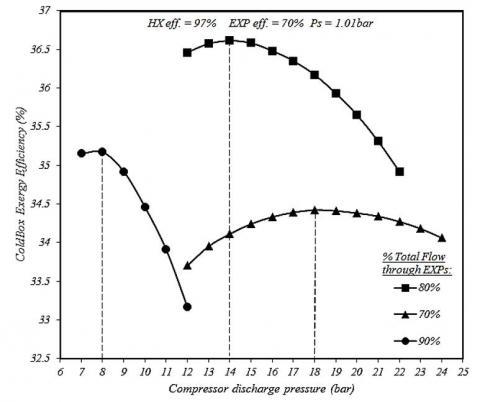Figure 8. Changes in the cold box exergy efficiency for various input flow rate to the expanders based on the compressor discharge pressure in the 4 expanders cycle

3.4 Effect of specific flow rate distribution between expanders

In this step, different flow rate distribution between expanders is investigated in order to obtain maximum efficiency at various pressures. The analysis is as follows: at every step, 10% of the flow rate of an expander is increased from the state, in which the total flow rate is divided equally between the expanders, and the same amount is reduced in the next step. During each change, level of flow rate for other expanders is assumed constant in order to precisely determine the importance of the input flow rate to each expander in the cycle. Figure 9 shows the simulation results for the 3 expander cycle.

• Similar to the dual-expander cycle, the maximum cold box exergy efficiency of the 3 expanders cycle belonged to the state that the flow rate is equally distributed among the expanders.
• The cycle optimal operating pressure is almost the same for various states of flow rate distribution and equaled 16 bar.
• In this figure, the curves for the flow rate distribution between the expanders are divided into two categories. The three lines marked with a rectangle show the state in which the fraction of input flow rate to the second and third expanders is increased, while the three lines marked with an ellipse indicate the state in which this flow rate is decreased. It is obvious that the cold box exergy efficiency is higher when this fraction increase compared with the state where it is reduced. This point reveals the significance of pre-cooling at the final stages of the cycle that may be due to the higher specific heat capacity of helium at lower temperatures of the cycle as well as the difference in flow rate among different parts of the cycle, especially the end of parts.
• The fraction of the input flow rate to the second expander is of special significance because the cold box exergy efficiency is higher when this fraction is increased in the second than the third expander. Moreover, the cold box exergy efficiency is higher when this fraction decreased in the second than the third expander.

## 9.jpg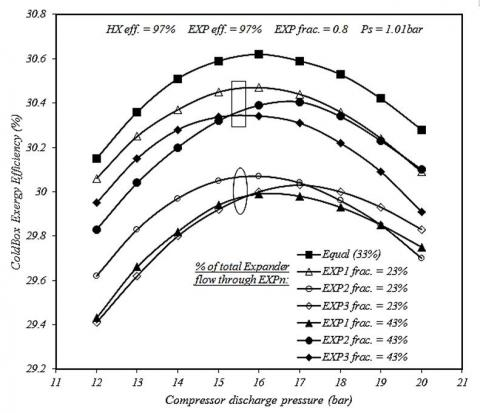Figure 9. Changes in the cold box exergy efficiency for the distribution of specific input flow rate among the expanders based on the compressor discharge pressure in the 3 expanders cycle

Figure 10 shows the simulation results for the 4 expander cycles.

• Similar to Collins and the 3 expanders cycle, the maximum exergy efficiency of the 4 expanders cycle belongs to the state that the flow rate is equally distributed among the expanders.
• The optimal operating pressure of the cycle is almost the same and equal to 14 bar for different states of flow rate distribution, except for the state in which the fourth expander flow rate is decreased by 10% (here, the optimal pressure is 19 bar). This issue indicates the importance and necessity of controlling the input flow rate to the fourth expander in the 4-expander cycle.
• In this figure, same to the 3 expanders cycle figure, the curves for the flow rate distribution among the expanders are divided into two categories. The four lines marked with a rectangle show the state in which the fraction of input flow rate to the second, third and fourth expanders is increased. The four lines marked with an ellipse indicate the state, in which this flow rate is decreased. It is obvious that the cold box exergy efficiency is higher when this fraction increases by comparing the state where it is reduced, further revealing the importance of pre-cooling at the final stages of the cycle.
• In the 4 expanders cycle, the fraction of the input flow rate to the third expander is specifically important because the cycle exergy efficiency is higher when this fraction is increased in the third expander than second and fourth expanders.

## 10.jpg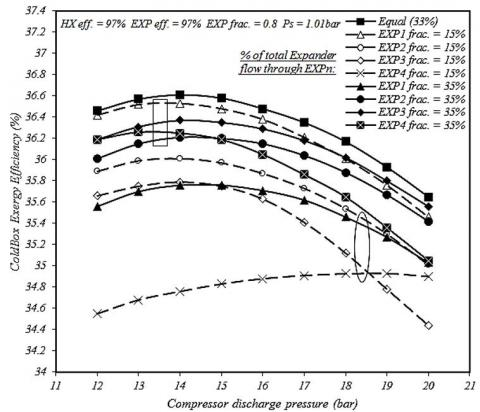Figure 10. Changes in the cold box exergy efficiency for the distribution of specific input flow rate among the expanders based on the compressor discharge pressure in the 4 expanders cycle

To further explain for the result of Figure 10, the chart of exergy destruction in the cycle equipment per input exergy to the 4 expanders cold box is drawn at the optimal pressure of 14 bar in Figure 11. Also, in Figure 12, the chart of hot flow temperature change along the cycle for the equal distribution of specific flow rate, as well as the limit states of the unequal specific flow rate, i.e. EXP1 frac.=15% and EXP4 frac.=15%, is presented. The following results are obtained:

• Based on the results of Figure 10, the exergy destruction is expected to be minimum for the equal distribution of specific flow rate and maximum for the EXP4 frac.=15% state, as correctly show in Figure 11.

## 11.jpgFigure 11. Exergy destruction ratio in different equipment of the liquefaction cycle to the total input exergy to the cold box in different states of the distribution of specific flow rate among the expanders of the 4 expanders cycle

• For other states, the exergy destruction is relatively equal. Therefore, to find the reason for the difference in the cold box exergy efficiency for these states, Figure 12 is drawn for the limit sates. It is clear that the hot flow temperature is decreased by different amounts in each cycle and at each pre-cooling stage. However, at the end stages, this temperature is almost equal in different cycles. Finally, the cycle, in which the distribution of specific flow rate is equal, had minimum input temperature to the cold end and, thus, the highest level of liquefaction and cold box exergy efficiency, because this cycle (i.e. equal distribution) consistently decrease the hot flow temperature over different pre-cooling stages.
• For the other two states, the difference is due to the temperature at the end of the cold box, with the results show in this figure.

## 12.jpgFigure 12. Changes in the temperature of the hot flow along the cycle in different states of the distribution of specific flow rate among the expanders of the 4 expanders cycle

3.5 Effect of heat exchangers effectiveness

In liquefaction cycles, such as helium liquefier, the heat exchangers effectiveness is highly important for achieving appropriate liquefaction. Figure 13 depicts the minimum effectiveness of the heat exchangers (assuming the same efficiency for all) of different liquefaction cycles for achieving minimum liquefaction. Compressor discharge pressure in this simulation, are considered optimal pressure of each cycle.

## 13.jpgFigure 13. Minimum effectiveness of the heat exchanger for generating liquid helium in different liquefaction cycles

Increasing the heat exchangers effectiveness must enhance the cold box exergy efficiency and liquefaction level because increasing the heat exchangers effectiveness leads to an increase in the level of heat transfer in it, thereby enhancing the cycle performance in pre-cooling of the hot flow (based on the constant flow rate of the cycle). For the 3 and 4 expander cycles, the effects of increasing the heat exchangers effectiveness on the cold box exergy efficiency based on the compressor discharge pressure are obtained in Figure 14 and Figure 15, respectively, with the following results:

• Increasing the heat exchangers effectiveness in all cycles significantly enhances the cold box exergy efficiency.
• Increasing the heat exchanger effectiveness decrease the cycle optimal operating pressure, thereby reducing the cycle required energy.
• Based on the results, the cycle liquefaction level is higher for the optimal state, but its operating pressure is less.

## 14.jpg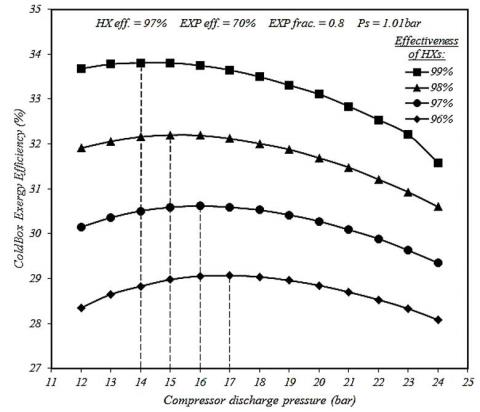Figure 14. Changes in the cold box exergy efficiency for the heat exchanger effectiveness based on the compressor discharge pressure in the 3 expanders cycle

## 15.jpg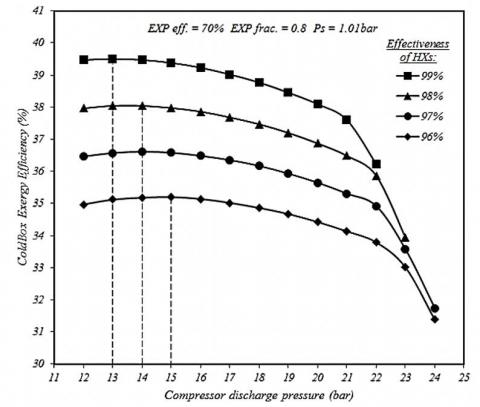Figure 15. Changes in the cold box exergy efficiency for the heat exchanger effectiveness based on the compressor discharge pressure in the 4 expanders cycle

Table 1, Table 2 and Table 3, respectively compare the cycle various properties at 96% and 99% heat exchanger effectiveness for the 2, 3 and 4 expander cycles. These tables indicate that:

• The positive effect of enhancing the heat exchanger effectiveness is higher in the cycles with fewer cooling stages. For example, increasing the heat exchanger effectiveness from 96% to 99% led to a change in the level of liquefaction in the cycle about 0.8% while this change is 0.5% for the 4 expander cycles with the same total input flow rate to the cold box.
• Similar to the level of liquefaction and the cold box exergy efficiency, the reduction on the cycle optimal pressure caused by increasing the heat exchanger effectiveness is higher in the cycle with fewer pre-cooling stages, showing the importance of the heat exchanger effectiveness in these cycles. As a result, the cycle optimal pressure does not remarkably change by increasing the heat exchanger effectiveness in the cycles with more pre-cooling stages.

Table 1. Comparing the cycle various properties at 96% and 99% HX effectiveness for the dual-expander cycle

 Popt (bar) ηcoldbox (%) y (%) y / Power [(kg/s) / (kW)] 2 EXP (99%) 16 24.81 6.266 15.02×10-6 2 EXP (96%) 22 19.42 5.475 11.47×10-6

Table 2. Comparing the cycle various properties at 96% and 99% HX effectiveness for the 3 expanders cycle

 Popt (bar) ηcoldbox (%) y (%) y / Power [(kg/s) / (kW)] 3 EXP (99%) 14 33.81 8.125 20.75×10-6 3 EXP (96%) 17 29.07 7.503 17.6×10-6

Table 3. Comparing the cycle various properties at 96 % and 99% HX effectiveness for the 4 expanders cycle

 Popt (bar) ηcoldbox (%) y (%) y / Power [(kg/s) / (kW)] 4 EXP (99%) 13 39.5 9.212 24.39×10-6 4 EXP (96%) 15 35.20 8.682 21.53×10-6

To further clarify the effect of increasing the heat exchanger effectiveness in helium liquefaction cycle, the chart of $(U A)^{*}$ of heat exchangers, e.g. for the 3 expanders cycle, is shown in Figure 16.

• For all the heat exchanger effectiveness, $(U A)^{*}$ of heat exchanger “1” is higher than other, this show the importance of this heat exchanger in helium pre-cooling.
• The value of $(U A)^{*}$ distributed from large to small is: HX1, HX3, HX2, HX4, HX5, HX6, and HX7.
• It is very important to note that, according to Table 3, it is observed that increasing the amount of liquefaction by changing heat exchangers effectiveness from 96% to 99% is approximately 0.6%, while with precision in Figure 16 it is observed that this change required double surface area. Therefore, the cycle manufacturer must consider the fact that the level of liquefaction is enhanced only by 0.6% through doubling the heat exchangers surface in the 3 expanders cycle.

## 16.jpg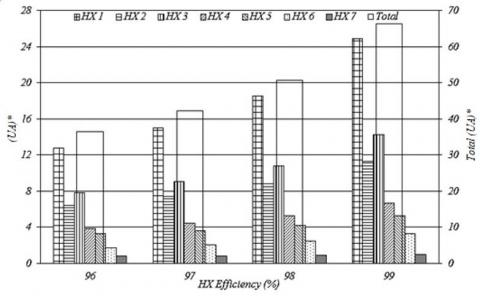Figure 16. Value of the non-dimensional heat transfer coefficient of heat exchangers based on their efficiency in the 3 expanders cycle

3.6 Effect of expanders efficiency

Similar to the effect of increasing the heat exchangers effectiveness on the cold box exergy efficiency, the pre-cooling of high-pressure helium flow is improved by enhancing the expanders efficiency, thereby increasing the cold box exergy efficiency and liquefaction level. For the 3 and 4 expander cycles, the effects of increasing the expanders efficiency based on the compressor discharge pressure are calculated in Figure 17 and Figure 18, respectively, with the following results:

• Increasing the expanders efficiency in all the cycles significantly enhances the cold box exergy efficiency. Increasing in the heat exchangers effectiveness requires the enhancement of the heat exchangers surface. Thus, in certain cases, the method of increasing the heat exchangers effectiveness could be replaced with that of enhancing the expanders efficiency for increasing cold box exergy efficiency.
• Increasing the expanders efficiency decrease the cycle optimal operating pressure, thereby reducing the cycle power consumption.
• Based on the results, the cycle liquefaction level is higher for the optimal state, in which the expander efficiency is higher, although its operating pressure is less.

## 17.jpgFigure 17. Changes in the cold box exergy efficiency in various expander efficiency based on the compressor discharge pressure in the 3 expanders cycle

## 18.jpg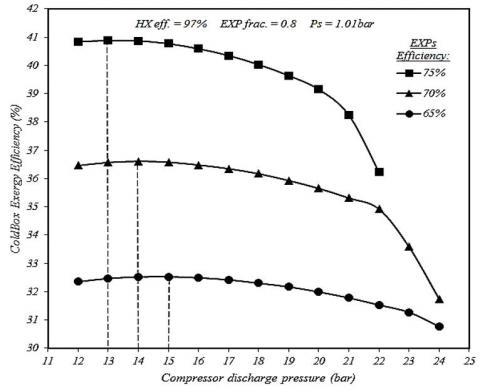Figure 18. Changes in the cold box exergy efficiency in various expander efficiency based on the compressor discharge pressure in the 4 expanders cycle

Same to the previous section, the effect of increasing the expanders efficiency in each cycle on the cold box exergy efficiency is studied by keeping the cycle high pressure constant. Results are presented in Figure 19.

## 19.jpgFigure 19. Changes in the cold box exergy efficiency for various stages in the liquefaction cycle based on the expander efficiency

• Similar to the effect of the heat exchanger effectiveness, increasing the expander efficiency in all the cycles significantly improve the cold box exergy efficiency.
• The changes in the cold box exergy efficiency based on the expander efficiency are almost linear. By using linear regression and calculating the slope of these lines, it is concluded that as the number of stages increase, the effect of expander efficiency on the cold box exergy efficiency increases (Contrary to the effect of heat exchanger effectiveness). This issue shows that in the cycles with numerous pre-cooling stages, increasing the expanders efficiency leads to a higher increase in the cold box exergy efficiency than increasing the heat exchangers effectiveness.

The effect of increasing the expander efficiency by 5% is higher on the cold box exergy efficiency and liquefaction level compare with the effect of increasing the heat exchanger efficiency by 2%. For example, for the 4 expanders cycle, increasing the expanders efficiency from 70% to 75% (i.e. 5% increase) results in the 3.5% increase in the cold box exergy efficiency and 0.95% increase in the liquefaction level. Nevertheless, increasing the heat exchanger effectiveness from 97% to 99% (i.e. by 2%) results in 2.44% increase in the cold box exergy efficiency and 0.66% increase in the liquefaction level.

4. Conclusions

Due to the unique properties of helium, it has been widely used in different applications which makes it a strategic material in the world. Most of the current large-scale helium Liquefaction industries are based on the Collins Liquefaction cycle. This cycle includes 3 parts namely compression, cooling, and Liquefaction. Each cooling stage has two heat exchangers and one expander. In this work, the effects of different parameters such as the number of cooling stage, discharge pressure of compressor unit, expander flow rate distribution, etc. on the performance of the Liquefaction cycle were investigated according to the exergy analyses.

The most important results of the present study are:

• Increasing the number of cycle pre-cooling stages enhance the cold box exergy efficiency from 22% (2 expanders cycle) to 36% (4 expanders cycle) at the optimal high pressure of every cycle.
• The highest exergy losses between the equipment of the cold box occur in the expanders. By increasing the pre-cooling stages, destruction will decrease in this equipment.
• Similar to the 2 expanders cycle, the highest exergy efficiency of the cold box in the 3 and 4 expander cycles occurs when the flow rate portion allocated to the expanders equaled 80% of the input flow rate to the cold box. Afterwards, in the 3 expanders cycle, the 70% flow rate ratio causes higher efficiency than the 90% flow rate ratio at the cycle optimal pressure point. Nevertheless, for the 4 expanders cycle, higher efficiency is achieved at the 90% expander flow rate ratio than the 70% one.
• For any input flow rate to the expanders which can determine the production conditions of the cycle and the utilized equipment, there is separate optimal pressure for the cycle. The cycle performance at this pressure provides the maximum output-to-input ratio.
• Similar to the Collins cycle, the maximum exergy efficiency of the cold box in 3 and 4 expander cycles has belonged to the state when the expanders flow rate is equally distributed among the expanders.
• The 3 and 4 expander cycles optimal operating pressure almost equaled 16 and 14 bar for various states of flow rate distribution.
• Similar to the level of liquefaction and the cold box exergy efficiency, the reduction on the cycle optimal pressure causes by increasing the heat exchanger effectiveness, is higher in the cycle with fewer pre-cooling stages, showing the importance of the heat exchanger effectiveness in these cycles. As a result, the cycle optimal pressure does not remarkably change by increasing the heat exchangers effectiveness in the cycles with high pre-cooling stages.
• The level of liquefaction is increased by approximately 0.6% by changing the heat exchanger effectiveness from 96% to 99%. However, based on the result, the total $(\mathrm{UA})^{*}$ for this change is double. Therefore, the cycle manufacturer must consider the fact that the level of liquefaction is enhanced only by 0.6% by doubling the exchanger’s dimension in the 3 expander cycles.
• As the number of stages in liquefaction cycle increases, the effect of heat exchangers effectiveness on the cold box exergy efficiency decreases. The changes in the cold box exergy efficiency based on the expander efficiency are almost linear. By using linear regression and calculating the slope of these lines, it is concluded that as the number of stages increases, the effect of expander efficiency on the cold box exergy efficiency increases (Contrary to the effect of heat exchanger effectiveness).
Nomenclature
 ${EX}^.$ Exergy rate, kW $Q^.$ heat transfer rate, kW $W^.$ work rate, kW $c_p$ specific heat, J. kg-1. K-1 $m^.$ Mass flow rate, kg .s-1 C.V control volume COMP compressor eff efficiency EXP Expander h Specific enthalpy, kJ. $\mathrm{kg}^{-1}$ HX Heat exchanger J-T Joule-Thomson valve s Specific entropy, kJ. $\mathrm{K}^{-1}$ SEP separator UA Overall heat transfer coefficient W/(m2K). (UA)* Non-dimensional heat transfer coefficient. A Heat transfer area (m2). Greek symbols Ψ exergy of flow per unit of mass, kJ kg-1 Δ difference $\eta_{e x}$ exergy efficiency Subscripts l liquid flow lp low-pressure comp compressor exp expander J-T Joule-Thomson valve flow flow dest destruction j each component 0 reference condition s suction g generation ex exergy
References

 Collins, S. (1947). A helium cryostat. Review of Scientific Instruments, 18(3): 157-67. https://doi.org/10.1103/physrev.70.98

 Mukhopadhyay, M. (2010). Fundamentals of Cryogenic Engineering. PHI Learning Pvt. Ltd.

 Thomas, R.J., Ghosh, P., Chowdhury, K. (2010). Thermodynamic analysis of collin’s cycle: Aspects of designing large scale helium liquefiers. In Proceedings of International Cryogenic Engineering Conference, 23: 291-297.

 Thomas, R.J., Ghosh, P., Chowdhury, K. (2011). Role of expanders in helium liquefaction cycles: Parametric studies using Collins cycle. Fusion Engineering and Design, 86(4): 318-24. https://doi.org/10.1016/j.fusengdes.2011.02.041

 Thomas, R.J., Basak, S., Ghosh, P., Chowdhury, K. (2008). Helium liquefaction/refrigeration system based on Claude cycle: A parametric study. In 22nd National Symposium on Cryogenics, Indian Institute of Science, Bangalore, India, pp. 1-4.

 Atrey, M. (1998). Thermodynamic analysis of Collins helium liquefaction cycle. Cryogenics, 38(12): 1199-206. https://doi.org/10.1016/S0011-2275(98)00110-6

 Trepp, C. (1962). Refrigeration systems for temperatures below 25 K with turboexpanders. Advances in Cryogenic Engineering, Springer Boston, MA, pp. 251-61. https://doi.org/10.1007/978-1-4757-0531-7_31

 Thomas, R.J., Ghosh, P., Chowdhury, K. (2012). Application of exergy analysis in designing helium liquefiers. Energy, 37(1): 207-19. https://doi.org/10.1016/j.energy.2011.11.044

 Thomas, R.J., Ghosh, P., Chowdhury, K. (2012). Exergy analysis of different cold end configurations for helium liquefiers. Journal of Thermal Science and Engineering Applications, 4(2): 021009. https://doi.org/10.1115/1.4005730

 Thomas, R.J., Ghosh, P., Chowdhury, K. (2013). Optimum number of stages and intermediate pressure level for highest exergy efficiency in large helium liquefiers. International Journal of Refrigeration, 36(8): 2438-57. https://doi.org/10.1016/j.ijrefrig.2013.06.008

 Matsubara, Y., Kaneko, M., Hiresaki, Y., Yasukochi, K. (1980). Exergy analysis of multi-staged Claude cycle helium refrigerator. Proceedings of cryogenic processes and equipment in energy systems conference San Fransisco: ASME of Conference Published.

 Ziegler, B. (1986). Second law analysis of the helium refrigerators for the HERA proton magnet ring. Advances in cryogenic engineering: Springer, 693-698. https://doi.org/10.1007/978-1-4613-2213-9_78

 Ziegler, B., Quack, H. (1992). Helium refrigeration at 40 percent efficiency? Adv Cryog Eng., 37: 645-51.

 Löhlein, K., Fukano, T. (1993). Exergy analysis of refrigerators for large scale cooling systems. Fusion Engineering and Design, 20: 511-518. https://doi.org/10.1016/0920-3796(93)90087-X

 Yu, J., Tian, G., Xu, Z. (2009). Exergy analysis of Joule–Thomson cryogenic refrigeration cycle with an ejector. Energy, 34(11): 1864-9. https://doi.org/10.1016/j.energy.2009.07.034

 Van der Ham, L., Kjelstrup, S. (2010). Exergy analysis of two cryogenic air separation processes. Energy, 35(12): 4731-9. https://doi.org/10.1016/j.energy.2010.09.019

 Remeljej, C., Hoadley, A. (2006). An exergy analysis of small-scale liquefied natural gas (LNG) liquefaction processes. Energy, 31(12): 2005-2019. https://doi.org/10.1016/j.energy.2005.09.005